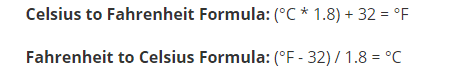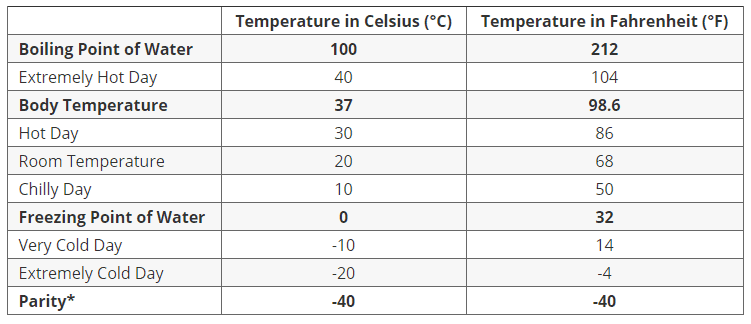How To Convert Celsius To Fahrenheit | Students Guide To Science

**Articles may contain links that I earn compensation for if clicked and you make a purchase. As an Amazon Associate, I earn from qualifying purchases. These earnings do not actually impact the price of the product or service.

When we study science, we often use the unit of the temperature In the Fahrenheit scale in America. But rest of the world (except two or three other countries), uses the Celsius scale. So, while we study some science books, we want to know How to Convert C To F. Do not worry I will give you all the answers of all your questions also you have examples, you can take them as a bonus. The formulas, and conversion. Hope they help you.Celsius vs Fahrenheit: Key Differences

Before I explain how to convert Celsius to Fahrenheit (and Fahrenheit to Celsius),

We should know the main difference between them, what they actually are.

• Celsius (written as °C and also called Centigrade) is the most common temperature scale around the globe, used by all but five continents.
• It’s part of the International System of Units (SI), or they are also known as the metric system, which is typically used in science (think centimeters, meters, kilograms, milliliters, etc.) and in science as a whole.
• Fahrenheit is part of the Imperial system, it includes forms of measurements such as inches, feet, pounds, gallons, etc. Moreover, unlike Celsius, it is not typically used in science or in the world except for a few places

Convert Celsius To Fahrenheit Formula

The How-To Convert C To F Formula for converting Celsius to Fahrenheit is:

F = 1.8 C + 32

We use F to symbolize Fahrenheit and C to symbolize celsius

The formula may also be written as:

F = 9/5 C + 32

Convert Celsius To Fahrenheit EquationBut you still want an easy way here are only two steps which you can use to convert them

1. Multiply your Celsius measurement by 1.8.
2. Add 32 to the result.

The final answer will be the temperature in Fahrenheit.

Note: If you are doing your homework do not forget to use the same numbers as given in the questions.

For Example-

While traveling in Europe you caught a fever. You only have access to a thermometer with Celsius measurements, which showed that your body temperature is 37degrees. You want to convert this measurement to Fahrenheit.

To do this, just put the values into the equation

F = 1.8 C + 32

F = (1.8)(37) + 32

F = 66.6 + 32

F = 98.6

The original value, 37 degrees Celsius, has two digits, so the Fahrenheit temperature should be approximately 99 degrees Fahrenheit.

Celsius To Fahrenheit Conversion Chart

Down below I am pasting a chart for conversion of the most commonly used temperatures in everyday life when converting from Celsius to Fahrenheit. You can  Memorize these and you will surely have an easier time converting from Celsius to Fahrenheit (and vice versa) fast.

Table of °C and °F Temperature Conversions

Here are some common important temperatures belowCelsius and Fahrenheit are two important temperature scales that are commonly misspelled as Celcius and Fahrenheit

Fun fact-The two temperature scales are equal at -40°.

Easy Way To Convert C To F

For Shortcut method –

For Example, let us say that the temperature outside is 18 °C and you want to know what this would equal in Fahrenheit. Just use the following equation-

(18 * 1.8) + 32

(32.4) + 32

=64.4 °F

Here is another example if you want to convert Fahrenheit to Celsius: let us say you’re feeling ill and your body temperature is 101.3 °F. To find out what this equals in Celsius, simply put the value 101.3 into the °F part in the second equation written above:

(101.3 – 32) / 1.8

(69.3) / 1.8

=38.5 °C

As can be seen, these conversions are not hard to carry out, but they do take some time and we can not solve them without using a calculator or a paper and pen.

But… I got a shortcut.you can just memorize the most common temperatures that are mostly used in daily life

How to Convert Celsius to Fahrenheit? A very Quick Trick

If you want to quickly convert Celsius to Fahrenheit, here is a simple trick you can use:

1. Multiply the temperature in degrees Celsius by 2
2. Add 30 in it to get the (estimated) temperature in degrees Fahrenheit.
3. The thumb rule is very useful and also almost accurate for most temperatures.

For example,

if the temperature outside is 15 °C, this would come out to about 60 °F:

(15 * 2) + 30

(30) + 30

= 60 °F (for real 15 °C is equivalent to 59 °F—that’s pretty close!)

Of course, expect the actual temperature to be a few degrees off, but for the most part, this is a reliable and easy way to convert temperatures in your head fast.

Do you want to convert Fahrenheit to Celsius? Just do the opposite:

1. Subtract 30 from the temperature in degrees Fahrenheit,
2. Divide by 2 to get the temperature in degrees Celsius.

For example,

if the temperature outside is 84 °F, this would be almost equal to 27 °C:

(84 – 30) / 2

54 / 2

= 27 °C (for real, 84 °F is equivalent to 28.89 °C—again, that’s a pretty close estimate!).

Conclusion

I hope you liked this article and if you really did please let me know at the comment section down below. And if you do not why? Please let me know the problem, I will surely reply and I will fix the problem. And also if you have other quarries about this topic you can also comment down below at the comment section, thank you for visiting, have a great day ahead.

Cover Image Credits

1 thought on “How To Convert Celsius To Fahrenheit | Students Guide To Science”

1.Do this for below zero Celsius conversions to Fahrenheit.